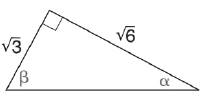Chapter 11.3, Problem 26EElementary Geometry For College St...

7th Edition
Alexander + 2 others
ISBN: 9781337614085

Solutions

Chapter
SectionElementary Geometry For College St...

7th Edition
Alexander + 2 others
ISBN: 9781337614085
Textbook Problem

In Exercises 21 to 26, use the sine, cosine, or tangent ratio to find the indicated angle measures to the nearest degree.To determine

To find:

The indicated angle measures to the nearest degree using the sine, cosine or tangent ratio.

Explanation

Given:

The triangle with indicated angles is shown below.

Definition used:

The trigonometric ratios:

Calculation:

Now consider the angle α.

From the figure,

tanα=36

α=tan1(36)

Let us use Ti-83 calculator to find the value of α.

Press ON in Ti-83 calculator.

Since we need to find the value of degree, we need to change the mode to degrees.

Press MODE button and use arrow keys to select the “Degree” mode.

Now press the following buttons in the same sequence.

2ndTAN2ndx2(3)÷2ndx2(6)

Then press ENTER

Still sussing out bartleby?

Check out a sample textbook solution.

See a sample solution

The Solution to Your Study Problems

Bartleby provides explanations to thousands of textbook problems written by our experts, many with advanced degrees!

Get Started

Find f. f(x) = 5x4 3x2 + 4, f(1) = 2

Single Variable Calculus: Early Transcendentals, Volume I

Expand each expression in Exercises 122. (3x+1)(2x2x+1)

Finite Mathematics and Applied Calculus (MindTap Course List)

find the real roots of each equation by factoring. 132. 6x2 + x + 12 = 0

Applied Calculus for the Managerial, Life, and Social Sciences: A Brief Approach

Explain how inter-rater reliability is established.

Research Methods for the Behavioral Sciences (MindTap Course List)